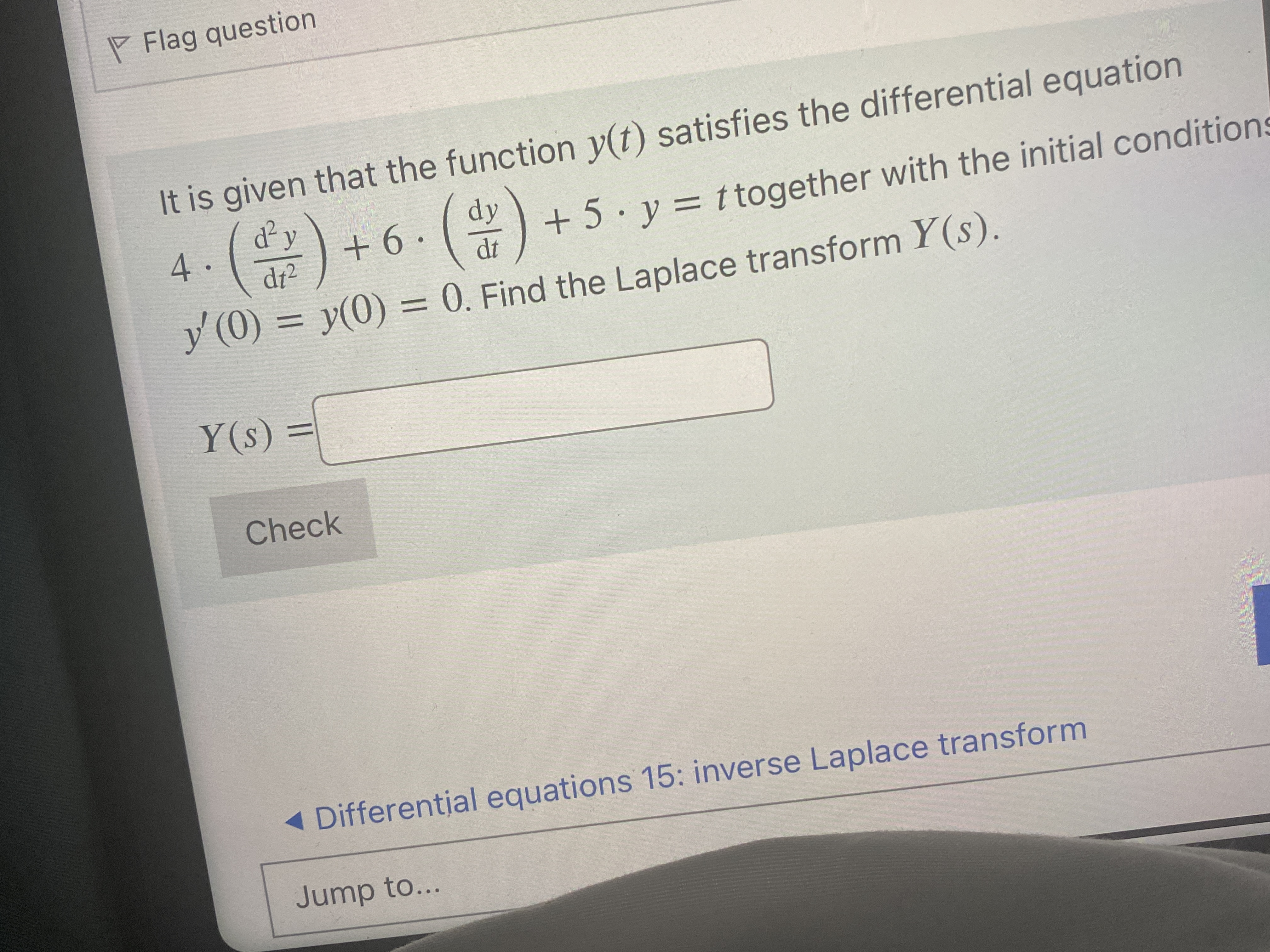# Get help with differential equations

Recent questions in Differential equations
2022-05-09

###estroishave4 2022-05-03 Answered

### Series solution of $x{y}^{{}^{″}}+2{y}^{{}^{\prime }}-xy=0$I get $r\left(r+1\right)=0,\left(r+1\right)\left(r+2\right){c}_{1}=0$ and${c}_{n+1}=\frac{{c}_{n-1}}{\left(n+1+r\right)\left(n+r+2\right)}$The first equation gives the indicial roots $r=-1$ and $r=0$. The case for $r=0$ is fine.For $r=-1$, I don't see how ${c}_{1}=0$ is implied by the second equation. The way I see it, since $1+r=0$ when $r=-1,{c}_{1}$ is not necessarily zero here, but this leads to a different solution to what is in the text book. What am I missing? Why is ${c}_{1}$ forced to be zero?sexe4yo 2022-05-02 Answered

### Second Order Differential Equations $ay{}^{″}+b{y}^{\prime }+cy=0$, without complex numbershapantad2j 2022-05-02 Answered

### Question in population dynamics using exponential growth rate equationGiven population doubles in 20 minutes, what is intrinsic growth rate r?Attempt: Given population doubles, using exponential growth rate we have $\frac{dN}{dt}=2N$ so $N\left(t\right)={N}_{0}{e}^{2t}$ therefore $r=2$, but I have a feeling this is wrong since 20 minutes should be used somewhere around here.Magdalena Norton 2022-05-02 Answered

### $\frac{dy}{dx}=y+1$ Solving the above given differential equation, yields the following general solution. $y+1={e}^{x+C}$$y=C{e}^{x}-1$$⇒$ Solution $y=-1$ at $C=0$Can I say that $y=-1$ is a singular solution?Sydney Stanley 2022-05-02 Answered

### $\left(1-{x}^{2}\right)y{}^{″}-x{y}^{\prime }+{m}^{2}y=0$, m is a constant. I have to show that $m\in \mathbb{Z}⇒deg\left(y\right)=m$ such that y is a solution of the ode.yert21trey123z7 2022-05-01 Answered

### Second order differential inequality and comparison theorem.If $x:\left[0,1\right]⇒\mathbb{R},x\in {C}^{\mathrm{\infty }}$ that satisfies$\left\{\begin{array}{l}\stackrel{¨}{x}\le -2x\\ x\left(0\right)=x\left(1\right)=0\end{array}$is it true that ?I observed that if $x\ge 0$, then $\stackrel{¨}{x}\le 0$, so x is concave. However I could not find out any other properties. I think if there exists a solution of the following differential equation$\left\{\begin{array}{l}\stackrel{¨}{x}=-2x\\ x\left(0\right)=x\left(1\right)=0\end{array}$then some kind of comparative theorem can be used. However, there is no non-trivial solution for this.If the above proposition is incorrect, could you give me a counterexample?hadaasyj 2022-05-01 Answered

### Prove instability using Lyapunov function${x}^{\prime }={x}^{3}+xy$${y}^{\prime }=-y+{y}^{2}+xy-{x}^{3}$pacificak8m 2022-05-01 Answered

### Linearization around the equilibrium point for a system of differential equations$\frac{dN}{dt}=\mu N\left(1-\frac{N}{K}\right)+N{\int }_{0}^{\mathrm{\infty }}p\left(a,t\right)da$$\frac{\partial p}{\partial t}+\frac{\partial p}{\partial a}=kp\left(a,t\right).$Linearizing it around $\left(N=K,p=0\right)$, I am getting the system$\frac{dN}{dt}=h-\mu N+N{\int }_{0}^{\mathrm{\infty }}p\left(a,t\right)da$$\frac{\partial p}{\partial t}+\frac{\partial p}{\partial a}=kp\left(a,t\right),$where $h=\mu K$.The reason I am getting this system is because I am considering $N{\int }_{0}^{\mathrm{\infty }}p\left(a,t\right)da$ as linear term. Is this linearisation right or the correct system is $\frac{dN}{dt}=h-\mu N$$\frac{\partial p}{\partial t}+\frac{\partial p}{\partial a}=kp\left(a,t\right),$where $h=\mu K$.Ali Marshall 2022-05-01 Answered

### ${x}^{2}y{}^{″}+\left(3{x}^{2}+4x\right){y}^{\prime }+2\left({x}^{2}+3x+1\right)y=0$I try to solve this equation by finding $\mu$ such that the equation become exact.( I know there are other ways for solving this equation).${\left(\mu {y}^{\prime }\right)}^{\prime }={\mu }^{\prime }{y}^{\prime }+\mu y{}^{″}=\mu y{}^{″}+\mu \frac{3{x}^{2}+4x}{{x}^{2}}{y}^{\prime }+\mu \frac{2\left({x}^{2}+3x+1\right)}{{x}^{2}}y⇒$${\mu }^{\prime }{y}^{\prime }=\mu \frac{3{x}^{2}+4x}{{x}^{2}}{y}^{\prime }+\mu \frac{2\left({x}^{2}+3x+1\right)}{{x}^{2}}y⇒\frac{{\mu }^{\prime }}{\mu }=\frac{3{x}^{2}+4x}{{x}^{2}}+\frac{2\left({x}^{2}+3x+1\right)}{{x}^{2}}\frac{y}{{y}^{\prime }}$How am I supposed to solve it?iyiswad9k 2022-05-01 Answered

### Proving single solution to an initial value problemor each $\left({x}_{0},{y}_{0}\right)\in \mathbb{R}×\mathbb{R}$ I need to prove that there is a single solution defined on $\mathbb{R}$Libby Boone 2022-05-01 Answered

### Proving single solution of initial value problem is increasingGiven the initial value problem${y}^{\prime }\left(x\right)=y\left(x\right)-\mathrm{sin}\left\{y\left(x\right)\right\},y\left(0\right)=1$Kaiya Hardin 2022-04-30 Answered

### Second Order Nonhomogeneous Differential Equation (Method of Undetermined Coefficients)Find the general solution of the following Differential equation $y{}^{″}-2{y}^{\prime }+10y={e}^{x}\mathrm{cos}\left(3x\right)$. We know that the general solution for 2nd order Nonhomogeneous differential equations is the sum of ${y}_{p}+{y}_{c}$ where ${y}_{c}$ is the general solution of the homogeneous equation and ${y}_{p}$ the solution of the nonhomogeneous. Therefore ${y}_{c}={e}^{x}\left({c}_{1}\mathrm{cos}\left(3x\right)+{c}_{2}\mathrm{cos}\left(3x\right)\right)$. Now we have to find ${y}_{p}$. I know in fact that ${y}_{c}={e}^{x}\left({c}_{1}\mathrm{cos}\left(3x\right)+{c}_{2}\mathrm{cos}\left(3x\right)\right)$. Now we have to find yp. I know in fact that ${y}_{p}={e}^{×}\frac{\mathrm{sin}\left(3x\right)}{6}$ but i do not know how to get there.adiadas8o7 2022-04-30 Answered

### Second-order ODE involving two functionsI am wondering how to find a general analytical solution to the following ODE:$\frac{dy}{dt}\frac{{d}^{2}x}{{dt}^{2}}=\frac{dx}{dt}\frac{{d}^{2}y}{{dt}^{2}}$The solution method might be relatively simple; but right now I don't know how to approach this problem.micelije1mw 2022-04-30 Answered

### Removal of absolute signsAn object is dropped from a cliff. The object leaves with zero speed, and t seconds later its speed v metres per second satisfies the differential equation$\frac{dv}{dt}=10-0.1{v}^{2}$So I found t in terms of v$t=\frac{1}{2}\mathrm{ln}|\frac{10+v}{10-v}|$The questions goes on like this: Find the speed of the object after 1 second. Part of the answer key shows this$t=\frac{1}{2}\mathrm{ln}|\frac{10+v}{10-v}|$$2t=\mathrm{ln}|\frac{10+v}{10-v}|$${e}^{2t}=\frac{10+v}{10-v}$So here's my question: why is it not like this?$±{e}^{2t}=\frac{10+v}{10-v}$Why can you ignore the absolute sign?Magdalena Norton 2022-04-30 Answered

### Question on a differential equationLet $x:\mathbb{R}⇒\mathbb{R}$ a solution of the differenzial equation:Proof that the function:$f:\mathrm{ℝ}⇒\mathrm{ℝ}$Maurice Maldonado 2022-04-30 Answered

### How to solve this nonlinear diff eq of celestial mechanics?${\left(\stackrel{˙}{r}\right)}^{2}=\frac{2\mu }{r}+2h$Where mu and h are constants.I have no idea how to solve it, maybe there is a trick I didn't know.The only thing that came in mind is to integrate$\int \frac{dr}{\sqrt{\frac{2\mu }{r}+2h}}=\int dt$but I don't think this is really a solution, since I don't know too how to evaluate the integral in terms of elementary functions.Elise Winters 2022-04-29 Answered

### Let u be a solution of the differential equation ${y}^{\prime }+xy=0$ and $\varphi =u\psi$ be a solution of the differential equation ${y}^{″}+2x{y}^{\prime }+\left({x}^{2}+2\right)y=0$ such that $\varphi \left(0\right)=1,{\varphi }^{\prime }\left(0\right)=0$. Then $\varphi \left(x\right)$ is equal toA) $\left({\mathrm{cos}}^{2}\left(x\right)\right){e}^{\frac{-{x}^{2}}{2}}$B) $\left(\mathrm{cos}\left(x\right)\right){e}^{\frac{-{x}^{2}}{2}}$C) $\left(1+{x}^{2}\right){e}^{\frac{-{x}^{2}}{2}}$D) $\left(\mathrm{cos}\left(x\right)\right){e}^{-{x}^{2}}$Alejandro Atkins 2022-04-29 Answered

### Let $f:\mathbb{R}⇒\mathbb{R}$ be a differentiable function, and suppose $f={f}^{\prime }$ and $f\left(0\right)=1$. Then prove $f\left(x\right)\ne 0$ for all $x\in \mathbb{R}$wuntsongo0cy 2022-04-29 Answered